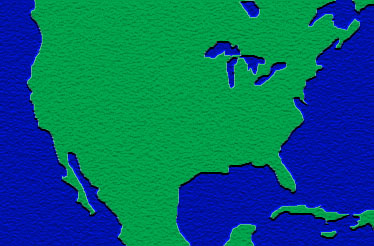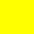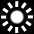Using An Alpha Channel This example shows how to matte one image onto another using an alpha channel. It also demonstrates a number of other useful techniques.
 1Creating the Background

First we create a Canvas and draw a background onto it. If we are happy with the Canvas having the same size as the image, we can use a shortcut and just pass the image file path directly to the Canvas constructor. This will create a Canvas, create a temporary XImage, set the Canvas size to the XImage size and draw the XImage on the Canvas.

[C#] ```Canvas canvas = new Canvas(Server.MapPath("rez/mapusa.jpg"));```

[Visual Basic] ```Dim canvas As New Canvas(Server.MapPath("rez/mapusa.jpg"))```

 2Creating the Foreground Image

Next we create the images we are going to draw onto our background. There are two images: the visible part and the alpha channel which defines a mask for the visible image. We insert the alpha channel into our visible image by calling SetChannel().

[C#] ```XImage picture = XImage.FromFile(Server.MapPath("rez/image.bmp")); picture.SetChannel("alpha", XImage.FromFile(Server.MapPath("rez/imagemask.bmp")));```

[Visual Basic] ```Dim picture As XImage = XImage.FromFile(Server.MapPath("rez/image.bmp")) picture.SetChannel("alpha", XImage.FromFile(Server.MapPath("rez/imagemask.bmp")))```

 3Drawing the Images

We are going to draw five images matted into the background. To do this we have to draw using transparent mode. Therefore we create DrawOptions by specifying true in the constructor. This ensures transparency will be used when drawing the images. Each image is offset 30 pixels down and 30 to the right from the top left. We use the Transform in the DrawOptions to achieve this. We end up with a diagonal line of images stretching down from the top left of the Canvas.

[C#] ```DrawOptions drawOpts = new DrawOptions(true); for (int i = 1; i <= 5; i++) { drawOpts.Transform.Translate(30, 30); canvas.DrawImage(picture, drawOpts); }```

[Visual Basic] ```Dim drawOpts = As DrawOptions(True); For i As Integer = 1 To 5 drawOpts.Transform.Translate(30, 30) canvas.DrawImage(picture, drawOpts) Next```

 4Saving

Finally we save the Canvas out as a file.

[C#] ```canvas.SaveAs(Server.MapPath("Using_An_Alpha_Channel_2.jpg")); ```

[Visual Basic] ```canvas.SaveAs(Server.MapPath("Using_An_Alpha_Channel_2.jpg")) ```

 5Input and Output

These are the kinds of input image you might use and the kind of output image you might expect.mapusa.jpgUsing_An_Alpha_Channel_2.jpgimage.bmp